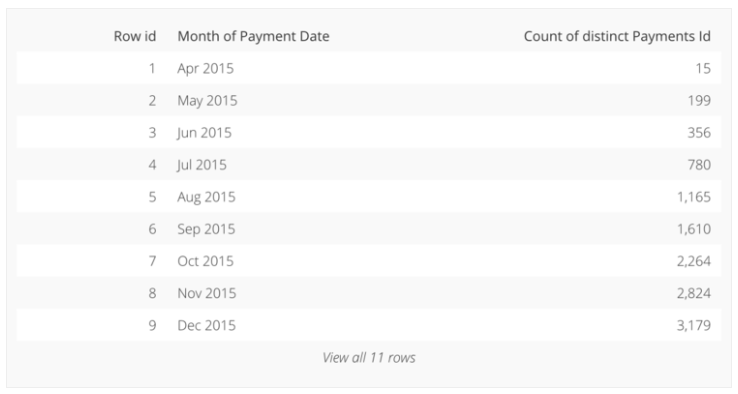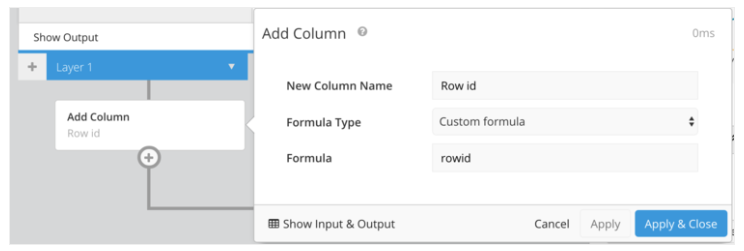# How to calculate Row Number using the Data Pipeline

Data Pipeline

Often, it can be handy to have row numbers when viewing data in tabular form. Let’s take a look at how we can calculate row numbers in the Data Pipeline in order to generate a table similar to the following:Open the chart in the Data Explorer, click the + button in the Data Pipeline, and choose Add Column.Choose “Custom formula” as our Formula Type, and enter the following into the Formula field:

``````rowid
``````If you want your Row id column to be the first column, add a Reorder Columns step.

See more